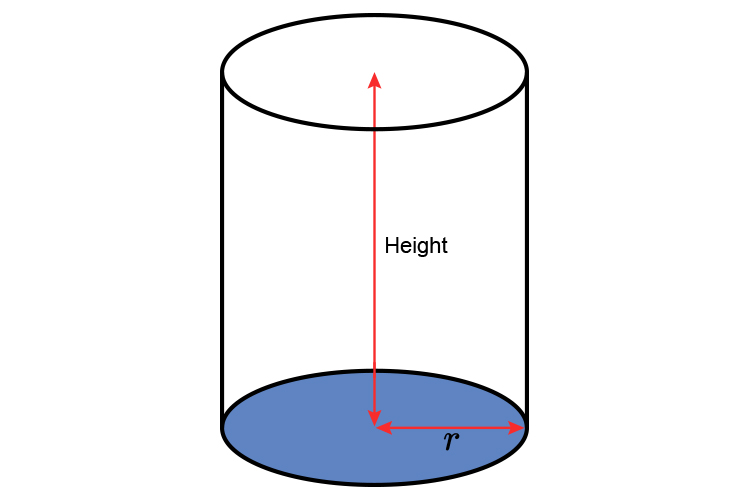# Volume of cylinders

The volume of a cylinder works on area of the base multiplied by height and some factor.Volume of cylinder = Area of base x height

Area of base = \pir^2

Therefore

Volume of a cylinder = \pir^2\times\ height